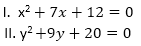Here, we are providing free crash course for LIC AAO Mains as there is left not enough time to deal in details. The questions asked in the quantitative aptitude section are calculative and very time-consuming. But once dealt with proper strategy, speed, and accuracy, this section can get you the maximum marks in the examination. Following is the Quantitative Aptitude quiz to help you practice with the best of latest pattern questions.

Q1. A invested Rs. X in a scheme. After 6 months, B joined with Rs. 4000 more than that of A. After an year, ratio of profit of B to the total profit was 3: 7. Find the value of X.
4000
8000
1600
6000
10000

Q2. 1500 rupees is invested in a scheme A at R% p.a. simple interest. Another amount (1500 - x) is invested in scheme B at 2R % p.a. simple interest. After 4 years, interest earned from scheme A is 25% less than that of scheme B. Find x.
1000
600
900
500
1200

Q3. 40 men can complete a work in 48 days. 64 men started for the same work for x days. After x days, 32 men increased, So, the remaining work is completed in 16(2/3) days. Find x.
5
8
10
6
None of these

Directions (4-8): What will come in place of (?) in the following number series problems? Q4. 200, 197, 185, 163, 130, ?
95
85
105
86
84

Q5. 15, 8, 9, 15, 32, ?
98
66
80.5
82.5
84.5

Q6. 5, 30, 150, 600, ?
1200
1500
2400
1800
600

Q7. 222, 110, 54, 26, ?
10
12
8
6
14

Q8. 104, ?, 96, 120, 88, 128
112
96
116
120
92

Directions (9-10): Solve the given quadratic equations and mark the correct option based on your answer.
Q9.if x>y
if x≥y
if x<y
if x ≤y
if x = y or no relation can be established between x and y.

Q10.if x>y
if x≥y
if x<y
if x ≤y
if x = y or no relation can be established between x and y.

Directions (11-15): Study the following graph carefully and answer accordingly:
The following graph shows the percentage profit of two companies over the years.Q11. The difference between the income of company Q in 2009 and that of P in 2004 was Rs. 405 lakhs, what was the difference in expenditure of Q in 2009 and that of P in 2004?
Rs 325 lakhs
Rs 350 lakhs
Rs 300 lakhs
Can’t be determined
None of these

Q12. In which of the following years is the ratio of expenditure to income for company Q the least (It is assumed that income of company Q is same in each year)?
2006
2003
2008
2005
2007
Solution:
Ratio of expenditure to income for company Q is the least when the percentage profit is Maximum. Hence, in 2006 the ratio was least.

Q13. If the income of company P in 2007 was equal to the expenditure of company Q in 2004, find the ratio of the income of company Q in 2004 to expenditure of company P in 2007.
8 : 15
5 : 6
6 : 5
15 : 8
3 : 5

Q14. If the income of company P in 2009 was Rs 660 lakhs, find the expenditure of company P in the same year.
Rs 600 lakhs
Rs 450 lakhs
Rs 400 lakhs
Data inadequate
None of these

Q15. If the income of company Q in 2005 was Rs 450 lakhs, find the expenditure of company Q in 2007.
Rs 300 lakhs
Rs 400 lakhs
Rs 675 lakhs
Can’t be determined
None of these
Solution:
Without knowing the income of Q for year 2007, the expenditure value can’t be determined

You May also like to Read: